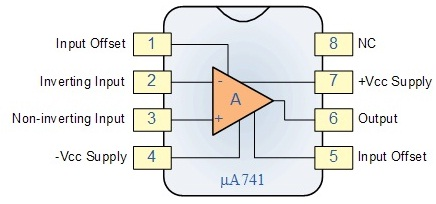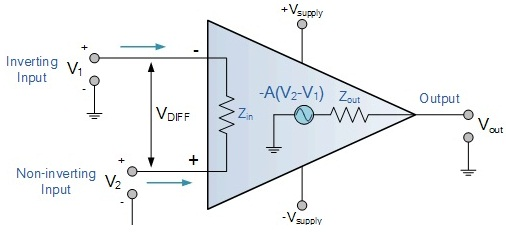Non - Inverting Amplifier

Objective

To design and study a Non - Inverting Amplifier using Op-amp 741.

Introduction

An electronic amplifier, amplifier, or (informally) amp is an electronic device that increases the power of a signal. It does this by taking energy from a power supply and controlling the output to match the input signal shape but with the larger amplitude. In this sense, an amplifier modulates the output of the power supply.

The second basic configuration of an operational amplifier circuit is that of a Non-inverting Amplifier. In this configuration, the input voltage signal, ( Vin ) is applied directly to the non-inverting (+) input terminal which means that the output gain of the amplifier becomes "Positive" in value in contrast to the "Inverting Amplifier" circuit we saw in the last tutorial whose output gain is negative in value. The result of this is that the output signal is "in-phase" with the input signal.

Feedback control of the non-inverting amplifier is achieved by applying a small part of the output voltage signal back to the inverting (-) input terminal via an Rƒ - R2 voltage divider network, again producing negative feedback. This closed-loop configuration produces a non-inverting amplifier circuit with very good stability, very high input impedance.

An operational Amplifier, often called an op-amp, is a DC-coupled high-gain electronic voltage amplifier with differential inputs ad usually a single output. Typically the output of the op-amp is controlled either by negative feedback, which largely determines the magnitude of its output Voltage gain, or by positive feedback, which facilitates regenerative gain and oscillation. High input impedance at the input terminals and low output impedance are important typical characteristics.

Ideal Op-amp: The figure below shows an example of an ideal operational amplifier. The main part in an amplifier is the dependent voltage source that increases in relation to the voltage drop across Rin, thus amplifying the voltage difference between V + and V -. Many uses have been found for Op-amp and an ideal Op-amp seeks to characterize the physical phenomena that make Op-amps useful.Fig.1 Op-amp 741Fig.2 Ideal Op-amp

Vs+ and Vs- are not connected to the circuit within the Op-amp because they power the dependent voltage source’s circuit. These are notable, however, because they determine the maximum voltage the dependent voltage source can output. For any input voltage the ideal Op-amp has,
a. Infinite open-loop gain
b. Infinite bandwidth
c. Infinite input impedance
d. Zero offset voltage
e. Infinite slew rate
f. Zero output impedance and
g. Zero noise